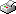Print This Page

Yuli Liang

-- licentiate thesis --

##### 'A Study of Multilevel Models with Block Circular Symmetric Covariance Structures

Abstract
This thesis concerns the study of multilevel models with specific patterned covariance structures and addresses the issues of maximum likelihood estimation. In particular, circular symmetric hierarchical data structures are considered.
In the first paper (Paper I), we consider so-called dihedral block symmetry models and extend them to models which covariance structures reflects both circularity and exchangeability present in the data. The main contribution of Paper I are two derived patterns of the covariance matrices which characterizes models under consideration. The relationship between these two patterned covariance matrices was investigated and it has been verified they are similar matrices. New expressions for the eigenvalues of block circular symmetric matrices are obtained which take into account the block structure. Paper II deals with estimation of balanced multilevel models with block circular symmetric covariance matrices. The spectral properties of such patterned covariance matrices are established. Maximum likelihood estimation is considered through the spectral decomposition of the patterned covariance matrix. The main results of Paper II concern the spectrum of the covariance matrix in the model of interest and the existence of explicit maximum likelihood estimators for the covariance parameters.

Keywords: Block circular symmetry, Covariance matrix, Explicit solution, Maximum likelihood estimator, Multilevel model, Spectrum.